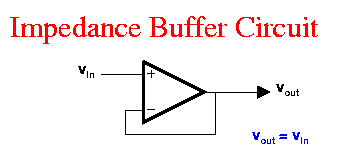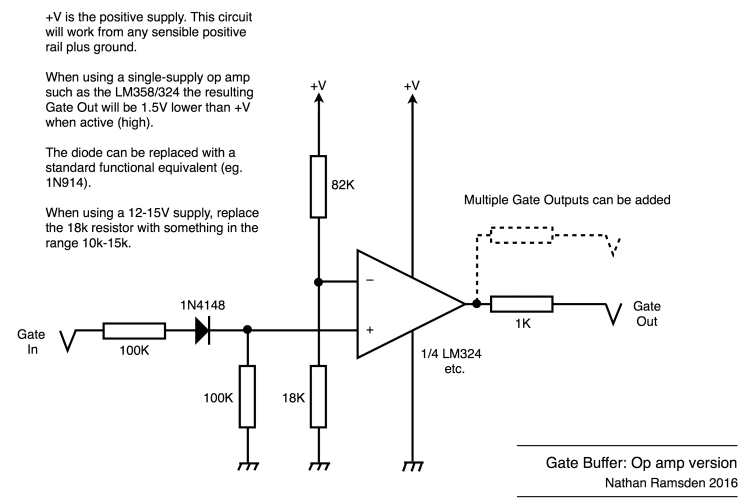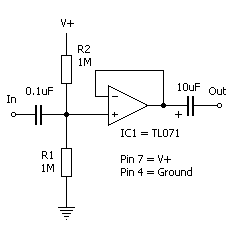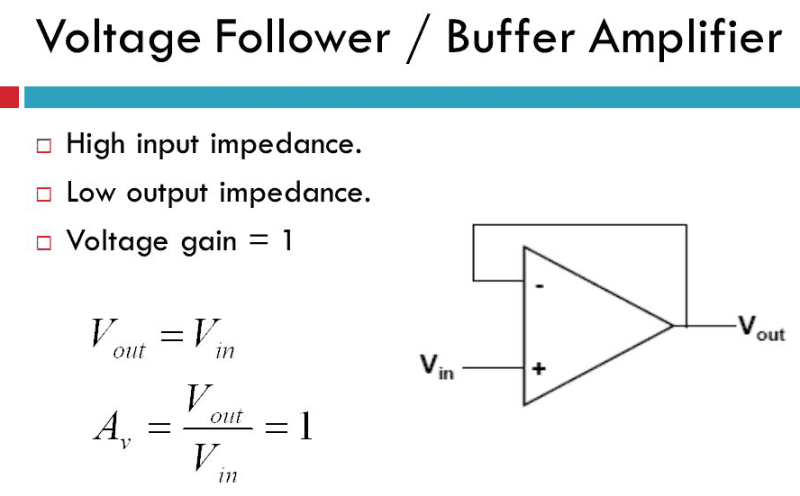# What's the advantage of using a voltage buffer amplifier?

RonzRknob
i want to understand whats the advantage of using a voltage follower

Voltux
A voltage follower is used to buffer the signal. It is helpful for changing impedance, too.

For example if you have a high impedance source that you cannot load, maybe a crystal oscillator, you can use the voltage follower to then distribute the signal to multiple loads, or maybe an amplifier that has a lower input impedance like a BJT.

•Asymptotic, sophiecentaur and davenn
Gold Member
2021 Award
i want to understand whats the advantage of using a voltage follower

A voltage follower is used to buffer the signal. It is helpful for changing impedance, too.

For example if you have a high impedance source that you cannot load, maybe a crystal oscillator, you can use the voltage follower to then distribute the signal to multiple loads, or maybe an amplifier that has a lower input impedance like a BJT.

great answer, Voltux@RonzRknob, a buffer, usually (but not always) has a gain of 1 ( unity gain) and is a great way to stop one circuit from affecting another

Using an op-amp in this configuration is a very popular practice eg …..The sole purpose of a buffer circuit is to isolate the input circuit from the output circuit

Dave

#### Attachments

•dlgoff and Asymptotic
RonzRknob
@davenn , can you further explain me why isolating the input circuit from the output circuit is beneficial?

Homework Helper
Gold Member
Imagine a source which has a series resistance of Rs and a load with resistance Rl. Try calculating how the power dissipated in the load changes as Rl changes. If Rl = 0 then there would be no voltage across it so the Power I*V would be zero. If RL was infinite there would be no current so again the power dissipated in the load would be zero. It so happens that the power is a maximum when Rl = Rs.

But what if they are fixed and RL <> Rs?

Inserting a buffer amplifier between the two allows you to change the impedance driving the load and maximise power transfer. This is what others mean by changing the impedance.

•RonzRknob
Gold Member
2021 Award
@davenn , can you further explain me why isolating the input circuit from the output circuit is beneficial?

I did answer that in post #3 as did @Voltux in post #2

His example of an oscillator circuit being affected ( loaded down) by the circuit placed on the output of the oscillator is an idea solution.
The circuit following the oscillator may be an amplifier stage, but just directly connection that amplifier to the output of the oscillator without any buffering isolation can cause the oscillator or function incorrectly, commonly by causing it to change it output frequency and or power output.
By putting a unity gain buffer stage in there between the oscillator and the amplifier, stops the amplifier stage from affecting the oscillators output. Thus the oscillator remains stable and its output doesn't frequency/power wobble all over the place.

RonzRknob
Imagine a source which has a series resistance of Rs and a load with resistance Rl. Try calculating how the power dissipated in the load changes as Rl changes. If Rl = 0 then there would be no voltage across it so the Power I*V would be zero. If RL was infinite there would be no current so again the power dissipated in the load would be zero. It so happens that the power is a maximum when Rl = Rs.

But what if they are fixed and RL <> Rs?

Inserting a buffer amplifier between the two allows you to change the impedance driving the load and maximise power transfer. This is what others mean by changing the impedance.
Thank you , that was helpful

•dlgoff
RonzRknob
I did answer that in post #3 as did @Voltux in post #2

His example of an oscillator circuit being affected ( loaded down) by the circuit placed on the output of the oscillator is an idea solution.
The circuit following the oscillator may be an amplifier stage, but just directly connection that amplifier to the output of the oscillator without any buffering isolation can cause the oscillator or function incorrectly, commonly by causing it to change it output frequency and or power output.
By putting a unity gain buffer stage in there between the oscillator and the amplifier, stops the amplifier stage from affecting the oscillators output. Thus the oscillator remains stable and its output doesn't frequency/power wobble all over the place.
Thanks Dave , I can now see it through

•dlgoff and davenn